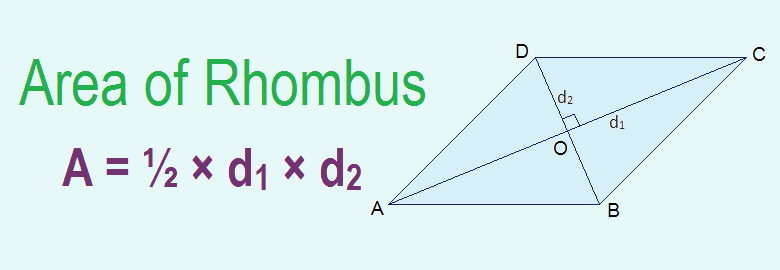# Area of Rhombus## Area of Rhombus

A rhombus is a quadrilateral having its all four sides equal. Area of rhombus is measured in terms of its two diagonals and is given by the half of the product of its two diagonals. i.e. Area of rhombus = ½ × d1 × d2, where d1 and d2 are two diagonals of rhombus.

### Derivation of the Formula for Area of Rhombus

Let, ABCD in the given figure be a rhombus. AC and BD are two diagonals which intersect each other at a point O.
We know that the diagonals of rhombus bisect each other at right angles.
OA = OC, OB = OD and AOB = BOC = COD = AOD = 90°
Let, BD = h1 and AC = h2
Now,
Area of the rhombus ABCD = area of ΔABD + area of ΔBCD
= ½ × BD × AO + ½ × BD × OC
= ½ × BD (AO + OC)
= ½ × BD × AC
= ½ × d1 × d2
Area of rhombus = ½ × d1 × d2, where d1 and d2 are two diagonals of rhombus.

### Perimeter of Rhombus

The perimeter of the rhombus = 4l, where l is the length of side of rhombus.

Workout Examples

Example 1: Find the area of the rhombus given below in the figure.
Solution: Here,
d1 = 8cm
d2 = 6cm
Area of rhombus = ½ ×d1× d2
= ½ × 8cm × 6cm
= 24cm2

You can comment your questions or problems regarding area of rhombus here.